# Part 7: Data Collection and Representation

In this article, we will explain data collection, and show you different types of data representation with worked examples.In this post, we provide a brief overview of what you will learn in Year 7 Data Collection and Representation.

Understanding Data Collection and Representation provides an important foundation in understanding Statistics: a significant part of future studies in both Maths and Science.

This article will introduce you to data and some of the many ways of representing data in various graphs and tables.

## Syllabus Outcomes

 Stage 4 NESA Maths; Statistics and Probability Syllabus Explanation Investigate techniques for collecting data, including census, sampling and observation (ACMSP284) This means that you can: Recognise variables as categorical or numerical (either discrete or continuous) Recognise and explain the difference between a ‘population’ and a ‘sample’ selected from a population when collecting data Construct and compare a range of data displays, including stem-and-leaf plots and dot plots (ACMSP170) This means that you can: Use a tally to organise data into a frequency distribution table Construct and interpret frequency histograms and polygons Construct dot plots Construct ordered stem-and-leaf plots, including stem-and-leaf plots with two-digit stems Construct divided bar graphs, sector graphs and line graphs, with and without the use of digital technologies Interpret a variety of graphs, including dot plots, stem-and-leaf plots, divided bar graphs, sector graphs and line graphs

## Data and statistics

Data is collected information which can then be analysed and interpreted to draw conclusions and inform our decisions.

Statistics is a branch of mathematics that encompasses the study of data.

There are two main types of data:

1. Categorical (or qualitative) data refers to qualities that can be placed into categories, or function as descriptive information.

Examples of categorical data include nationality and gender, as these qualities can be placed in clear categories.

This data is descriptive and cannot be expressed by numbers.

Nationality can be divided into “Australian”, “Belgian”, “Chilean” and other categories, but nationality cannot be represented by numbers such as $$54$$ or $$37.23$$.

2. Numerical (or quantitative) data refers to information that can be represented by numbers.

Examples of numerical data include height and weight.

Numerical data can be further divided into two groups:

• Discrete data is numerical data that is collected by counting exact values. Examples of discrete data include class enrolments. The data can only be whole numbers.
• Continuous data is numerical data that is collected by measuring and uses approximate values. Examples of continuous data include height and time. Data of this form cannot be measured exactly, but it is not limited to whole number quantities.

### Data collection

Data can be collected in many ways, including surveys, questionnaires and even direct measurement.

A real-world example of data collection is the national census.

The census collects data such as age, gender, incomes, occupations and much more to provide an understanding of the Australian populace.

Participation in the census is compulsory, which ensures all Australians are included.

In contrast to large-scale compulsory data collection like the national census, sample surveys collect data from a small sample of the population.

Such surveys are cheaper and easier to conduct.

However, statisticians must ensure the selected sample is representative of the entire population to give a reliable snapshot of the population.

Participants are often selected randomly to avoid surveying a sample that is not representative of the general population.

Collected data needs to be organised and represented in graphical or tabular form.

Then, it can be analysed to draw conclusions.

## Refine your child’s Maths skills

Join over 4500 students and build strong foundational Maths skills for senior success! Book a free trial now.## Data representation

### Frequency Distribution Tables

Frequency distribution tables are a common way of organising and representing data.

• The first column lists the categories or numerical scores.
• The second column is usually used to tally, or add-up, the collected data. This helps us to organise an unsorted dataset.
• The third column contains the frequency of the category/score, which is the sum of the tally marks.
• At the bottom of the frequency column, we display the sum of frequencies $$(\Sigma f)$$.

For example,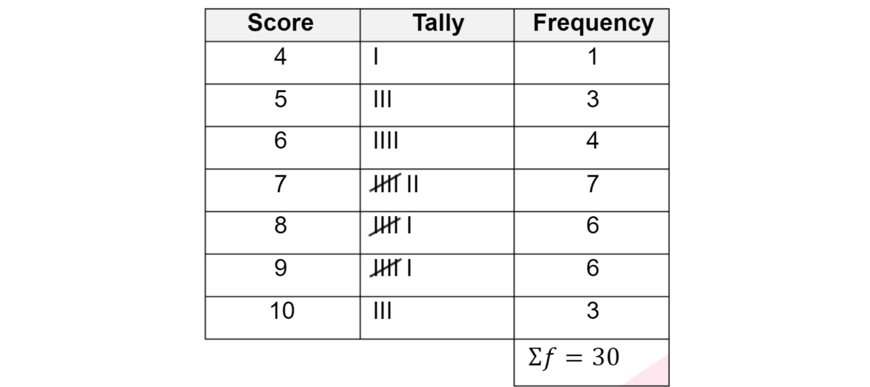Example

1. A Year 7 Matrix class had the following scores in their weekly quiz:

\begin{align*}
5, 3, 4, 2, 1, 5, 4, 5, 2, 3, 1, 0, 5, 3, 4, 3
\end{align*}

Copy and fill out the following frequency distribution table with the above data.

Then, identify the most common score(s).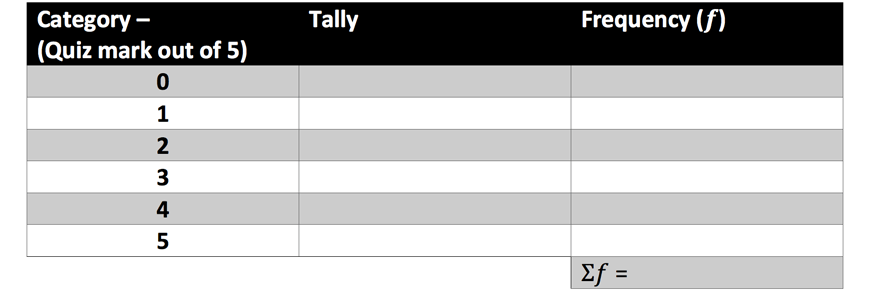Solution: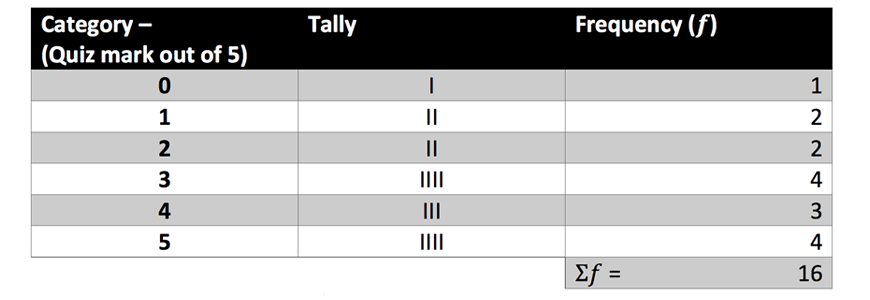The most common scores are $$3$$ and $$5$$.

### Frequency histograms and polygons

Data can also be represented in graphs.

Data organised in a frequency distribution table can produce two types of graph:

• Frequency histograms are a type of column graph which has no spaces between adjacent columns.
• The categories/scores are displayed on the horizontal axis.
• The frequency is displayed on the vertical axis.
• Frequency polygons are a type of line graph which usually shares a set of axes as a frequency histogram.

Frequency histograms and polygons are suitable for representing numerical data, or categorical data that can be ordered

E.g. age ranges: $$0-10, 11-20$$ etc.

Key features in frequency histograms and polygons:

• Clearly labelled $$x-axis$$ (Score) and $$y-axis$$ (Frequency).
• A title to define the graph.
• Each column of the histogram must be centred on the markings on the $$x-axis$$.
• They must be exactly $$1$$ unit in width
• The first column must be $$\frac{1}{2}$$ a unit from the $$y-axis$$.
• The polygon must start at the origin and pass through the midpoint of the tops of each column.
• The polygon must end on the $$x-axis$$, a $$\frac{1}{2}$$ unit away from the end of the last column.

Eg. The following graph is an example of a frequency histogram and frequency polygon on the same set of axes.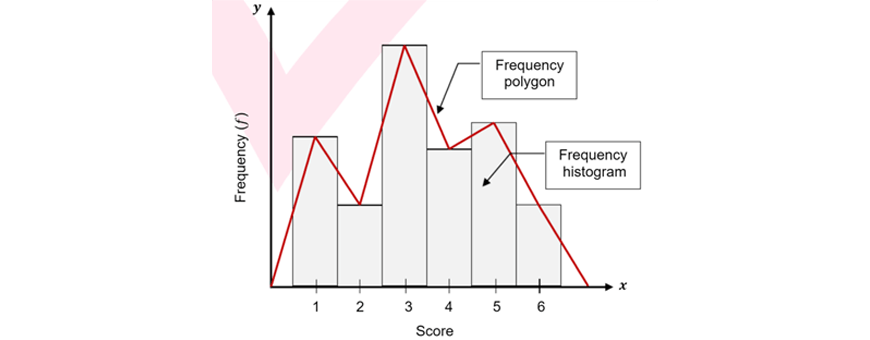Example

1. On the same set of axes, draw a frequency histogram and a frequency polygon for the following dataset: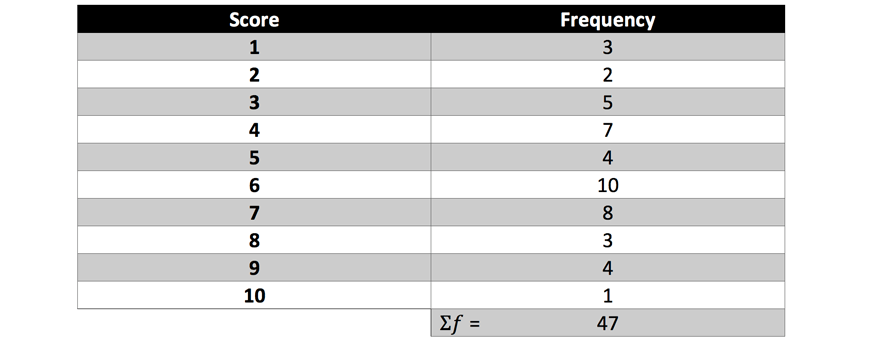Solution: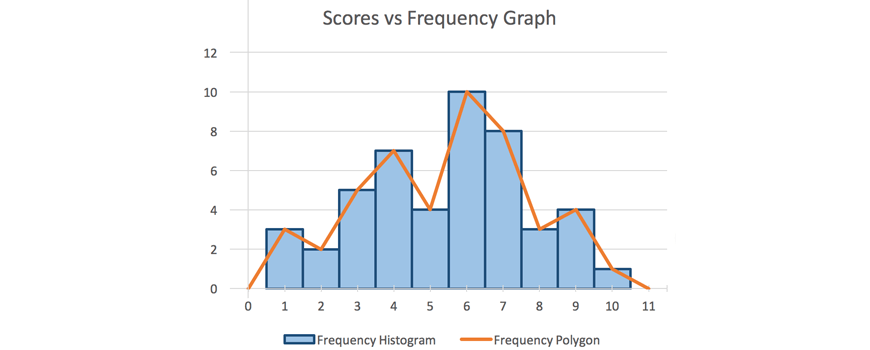### Dot plots

A dot plot is a graph that looks like columns of dots stacked on top of each other.

Dot plots can organise and display small sets of unsorted data.

However, they can be time-consuming to read or produce for large sets of data.

• The horizontal scale is a number line of scores or categories.
• There is no vertical scale. Dots are stacked evenly on top of each other.
• Stacks of dots are useful for showing relative frequencies of scores and where scores cluster.

For example, The following graph is an example of a dot plot.Each dot represents a score. All dots are spaced evenly so that the heights of the columns of dots represent the frequency of that score.

Example

1. A group of children are playing in a playground. Each was asked their age. Organise the following dataset in a dot plot:

\begin{align*}
10, 7, 9, 11, 9, 8, 10, 7, 10
\end{align*}

Solution:Note: your number line does not need to start at $$0$$!

### Sector graphs

Sector graphs (also known as pie charts) are circular graphs that are divided into sectors to represent the relative frequency of scores.

Sector graphs are useful for representing percentages or fractions.

• The whole circle represents the total sample.
• The area of each sector represents how much of the total sample gave a particular score.

Sector graphs are most suited to representing categorical data.

Dividing up the graph into too many sectors would be messy and unreadable.

For example, the following sector graph represents favourite sports of year 7 students.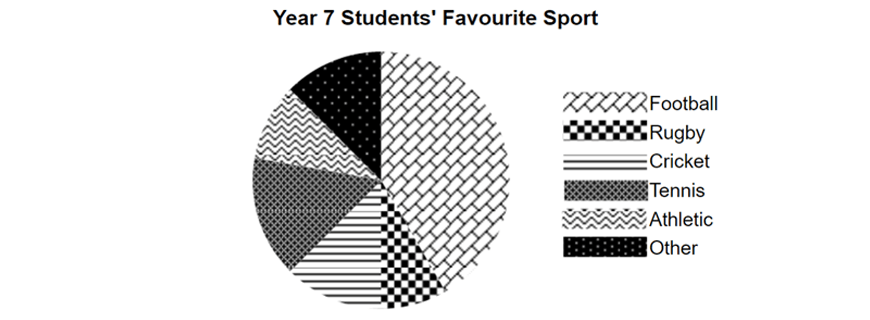Sector graphs can easily show which categories are more popular compared to others by comparing the area of sectors.

Example

1. A Matrix class was asked what their favourite pet was. Complete the following table and draw a sector graph to represent the following data: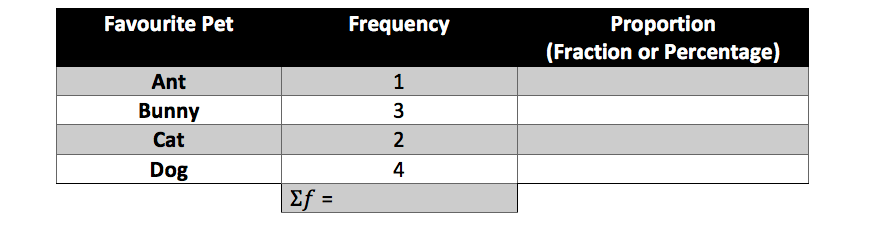Solution: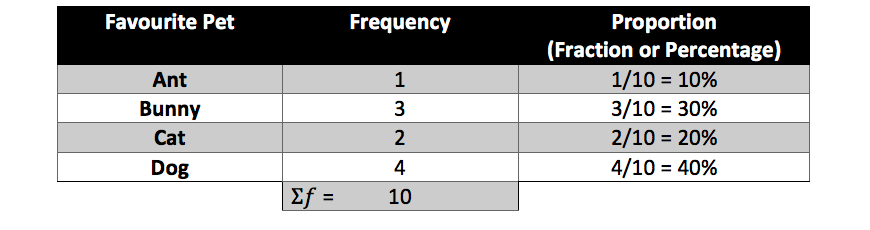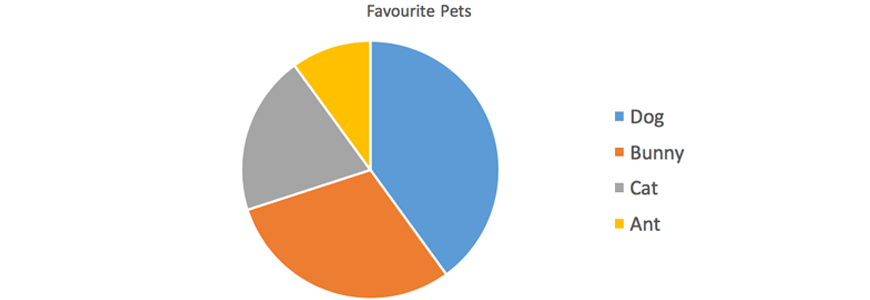### Bar and divided bar graphs

Bar graphs use bars of different heights to display data. A frequency histogram is one type of bar graph.

• The horizontal axis represents the scores and the vertical axis represents the frequency of scores.
• Bar graphs are well-suited to represent categorical data. However, they can also represent numerical data.
• Frequency histograms require much stricter formatting than ordinary bar graphs.
• Ordinary bar graphs can have spaces between bars and do not need to be strictly centred on horizontal axis markings.

Divided bar graphs represent proportions, or relative frequencies, of scores like sector graphs.

• Instead of a circle divided into sectors, the data is represented by a bar divided into sections.
• The bar represents the total sample.
• The area of each section represents how much of the total sample gave a particular score.
• Like sector graphs, divided bar graphs are more suited to representing categorical data.

For example, the following divided bar graph shows favourite fast foods.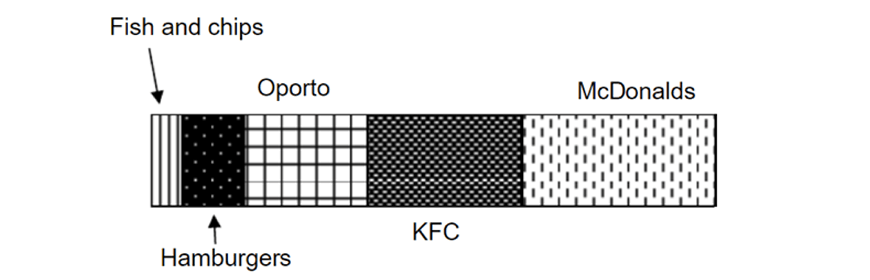Divided bar graphs also easily show which categories are more popular compared to others by looking at the lengths or areas of different sections

Example

1. Some Year 7 students were asked what their favourite fruit was. Complete the following table and construct a divided bar graph to represent the data.Solution: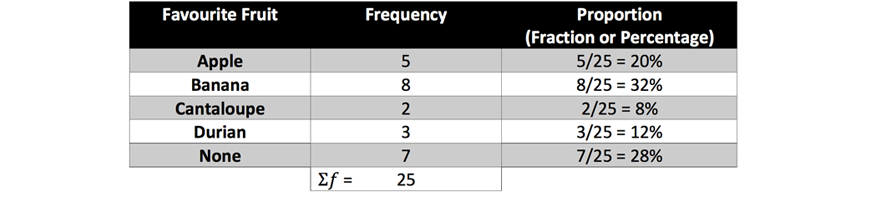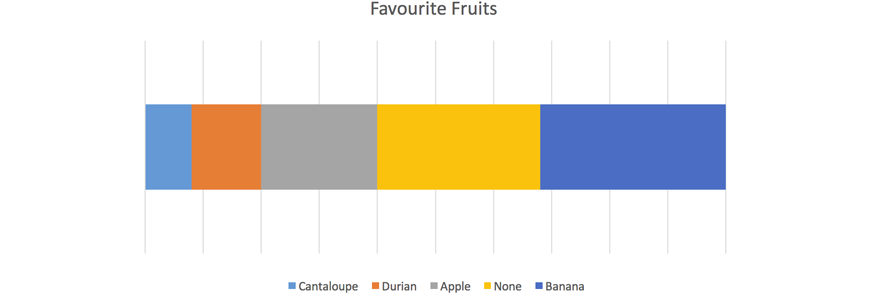### Stem and leaf plots

Stem and leaf plots are a tabular way of representing numerical data.

Stem and leaf plots look like a column and rows of digits.

• The table is divided into two columns – the stem column and the leaf
• The stem column lists each of the ‘tens’ digit in ascending order
• The leaf column lists the ‘ones’ digit of each score as a row in the column. These branch out from the stem in ascending order.
• Every score in a given dataset is represented by its own leaf.
• Not every stem has a leaf – some stems may be empty.

For example,

The following data:

\begin{align*}
7, 20, 32, 37, 37, 40, 40, 43, 47, 49, 49, 49, 50
\end{align*}

Would produce the following stem and leaf plot:Note: the $$‘1 \ stem’$$ has no leaves and is empty, whereas the $$‘0’$$ in the $$‘2 \ stem’$$ refers to the score $$‘20’$$.

Furthermore, each row of leaves must be in order, even if the data set you are given is unordered.

Example

1. Given this unordered data set:

\begin{align*}
45, 23, 24, 34, 26, 38, 30, 44, 10, 41, 20, 30, 41, 37
\end{align*}

Construct an ordered stem and leaf plot.

(Hint: first sort data by the $$‘tens’$$ digit)

Solution:

Sorting by the $$‘tens’$$ digits gives us:

\begin{align*}
&10 \\
&23, 24, 26, 20 \\
&34, 38, 30, 30, 37 \\
&45, 44, 41, 41
\end{align*}

We can then organise the data in a stem and leaf plot:## Summary

From this post, you have learnt:

• Data is information we can analyse and interpret to draw conclusions though the study of statistics.
• Data can be divided into numerical and categorical data.
• Numerical data can be divided into discrete and continuous data.

• Data can be collected in various ways:
• A national census collects data from all Australians to provide information about our population.
• Sample surveys are a small-scale way of collecting data from a smaller sample to represent a larger population.
• Samples must be chosen randomly to avoid bias and to properly represent a larger population.

• Various graphs and tables can be used to organise and represent data.
• Different types of data representation are more suitable for different types of data.
• Properly representing data helps us understand and use the data to draw conclusions.

© Matrix Education and www.matrix.edu.au, 2023. Unauthorised use and/or duplication of this material without express and written permission from this site’s author and/or owner is strictly prohibited. Excerpts and links may be used, provided that full and clear credit is given to Matrix Education and www.matrix.edu.au with appropriate and specific direction to the original content.